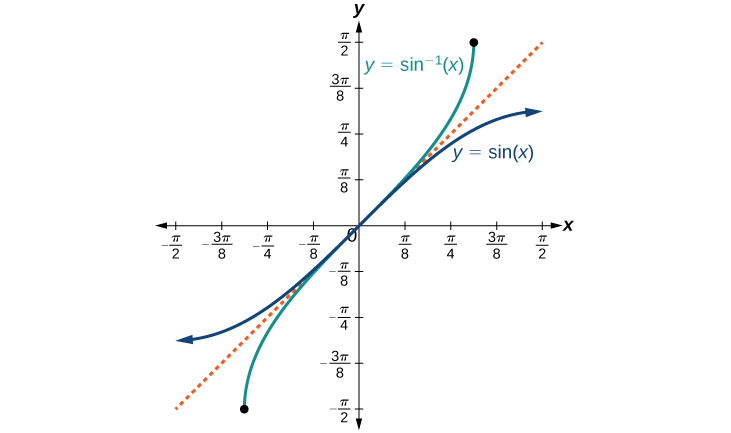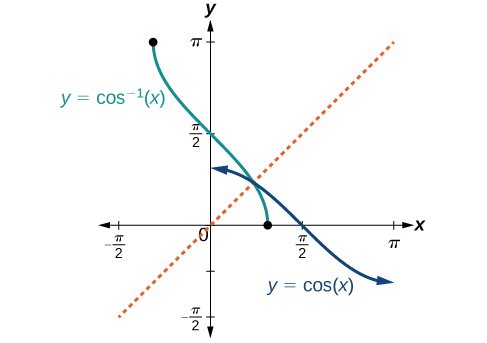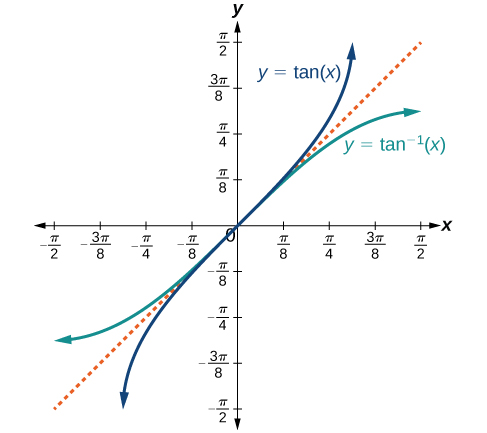# 8.3 Inverse trigonometric functions  (Page 2/15)

 Page 2 / 15

On these restricted domains, we can define the inverse trigonometric functions .

• The inverse sine function     $\text{\hspace{0.17em}}y={\mathrm{sin}}^{-1}x\text{\hspace{0.17em}}$ means $\text{\hspace{0.17em}}x=\mathrm{sin}\text{\hspace{0.17em}}y.\text{\hspace{0.17em}}$ The inverse sine function is sometimes called the arcsine    function, and notated $\text{\hspace{0.17em}}\mathrm{arcsin}x.$
• The inverse cosine function     $\text{\hspace{0.17em}}y={\mathrm{cos}}^{-1}x\text{\hspace{0.17em}}$ means $\text{\hspace{0.17em}}x=\mathrm{cos}\text{\hspace{0.17em}}y.\text{\hspace{0.17em}}$ The inverse cosine function is sometimes called the arccosine    function, and notated $\text{\hspace{0.17em}}\mathrm{arccos}\text{\hspace{0.17em}}x.$
• The inverse tangent function     $\text{\hspace{0.17em}}y={\mathrm{tan}}^{-1}x\text{\hspace{0.17em}}$ means $\text{\hspace{0.17em}}x=\mathrm{tan}\text{\hspace{0.17em}}y.\text{\hspace{0.17em}}$ The inverse tangent function is sometimes called the arctangent    function, and notated $\text{\hspace{0.17em}}\mathrm{arctan}\text{\hspace{0.17em}}x.$

The graphs of the inverse functions are shown in [link] , [link] , and [link] . Notice that the output of each of these inverse functions is a number, an angle in radian measure. We see that $\text{\hspace{0.17em}}{\mathrm{sin}}^{-1}x\text{\hspace{0.17em}}$ has domain $\text{\hspace{0.17em}}\left[-1,1\right]\text{\hspace{0.17em}}$ and range $\text{\hspace{0.17em}}\left[-\frac{\pi }{2},\frac{\pi }{2}\right],$ ${\mathrm{cos}}^{-1}x\text{\hspace{0.17em}}$ has domain $\text{\hspace{0.17em}}\left[-1,1\right]\text{\hspace{0.17em}}$ and range $\text{\hspace{0.17em}}\left[0,\pi \right],\text{\hspace{0.17em}}$ and $\text{\hspace{0.17em}}{\mathrm{tan}}^{-1}x\text{\hspace{0.17em}}$ has domain of all real numbers and range $\text{\hspace{0.17em}}\left(-\frac{\pi }{2},\frac{\pi }{2}\right).\text{\hspace{0.17em}}$ To find the domain    and range    of inverse trigonometric functions, switch the domain and range of the original functions. Each graph of the inverse trigonometric function is a reflection of the graph of the original function about the line $\text{\hspace{0.17em}}y=x.$The sine function and inverse sine (or arcsine) functionThe cosine function and inverse cosine (or arccosine) functionThe tangent function and inverse tangent (or arctangent) function

## Relations for inverse sine, cosine, and tangent functions

For angles in the interval $\text{\hspace{0.17em}}\left[-\frac{\pi }{2},\frac{\pi }{2}\right],\text{\hspace{0.17em}}$ if $\text{\hspace{0.17em}}\mathrm{sin}\text{\hspace{0.17em}}y=x,\text{\hspace{0.17em}}$ then $\text{\hspace{0.17em}}{\mathrm{sin}}^{-1}x=y.$

For angles in the interval $\text{\hspace{0.17em}}\left[0,\pi \right],\text{\hspace{0.17em}}$ if $\text{\hspace{0.17em}}\mathrm{cos}\text{\hspace{0.17em}}y=x,\text{\hspace{0.17em}}$ then $\text{\hspace{0.17em}}{\mathrm{cos}}^{-1}x=y.$

For angles in the interval $\text{\hspace{0.17em}}\left(-\frac{\pi }{2},\frac{\pi }{2}\right),\text{\hspace{0.17em}}$ if $\text{\hspace{0.17em}}\mathrm{tan}\text{\hspace{0.17em}}y=x,\text{\hspace{0.17em}}$ then $\text{\hspace{0.17em}}{\mathrm{tan}}^{-1}x=y.$

## Writing a relation for an inverse function

Given $\text{\hspace{0.17em}}\mathrm{sin}\left(\frac{5\pi }{12}\right)\approx 0.96593,\text{\hspace{0.17em}}$ write a relation involving the inverse sine.

Use the relation for the inverse sine. If $\text{\hspace{0.17em}}\mathrm{sin}\text{\hspace{0.17em}}y=x,\text{\hspace{0.17em}}$ then $\text{\hspace{0.17em}}{\mathrm{sin}}^{-1}x=y$ .

In this problem, $\text{\hspace{0.17em}}x=0.96593,\text{\hspace{0.17em}}$ and $\text{\hspace{0.17em}}y=\frac{5\pi }{12}.$

${\mathrm{sin}}^{-1}\left(0.96593\right)\approx \frac{5\pi }{12}$

Given $\text{\hspace{0.17em}}\mathrm{cos}\left(0.5\right)\approx 0.8776,$ write a relation involving the inverse cosine.

$\mathrm{arccos}\left(0.8776\right)\approx 0.5$

## Finding the exact value of expressions involving the inverse sine, cosine, and tangent functions

Now that we can identify inverse functions, we will learn to evaluate them. For most values in their domains, we must evaluate the inverse trigonometric functions by using a calculator, interpolating from a table, or using some other numerical technique. Just as we did with the original trigonometric functions, we can give exact values for the inverse functions when we are using the special angles, specifically $\text{\hspace{0.17em}}\frac{\pi }{6}\text{\hspace{0.17em}}$ (30°), $\text{\hspace{0.17em}}\frac{\pi }{4}\text{\hspace{0.17em}}$ (45°), and $\text{\hspace{0.17em}}\frac{\pi }{3}\text{\hspace{0.17em}}$ (60°), and their reflections into other quadrants.

Given a “special” input value, evaluate an inverse trigonometric function.

1. Find angle $\text{\hspace{0.17em}}x\text{\hspace{0.17em}}$ for which the original trigonometric function has an output equal to the given input for the inverse trigonometric function.
2. If $\text{\hspace{0.17em}}x\text{\hspace{0.17em}}$ is not in the defined range of the inverse, find another angle $\text{\hspace{0.17em}}y\text{\hspace{0.17em}}$ that is in the defined range and has the same sine, cosine, or tangent as $\text{\hspace{0.17em}}x,$ depending on which corresponds to the given inverse function.

## Evaluating inverse trigonometric functions for special input values

Evaluate each of the following.

1. ${\text{sin}}^{-1}\left(\frac{1}{2}\right)$
2. ${\text{sin}}^{-1}\left(-\frac{\sqrt{2}}{2}\right)$
3. ${\mathrm{cos}}^{-1}\left(-\frac{\sqrt{3}}{2}\right)$
4. ${\mathrm{tan}}^{-1}\left(1\right)$
1. Evaluating $\text{\hspace{0.17em}}{\mathrm{sin}}^{-1}\left(\frac{1}{2}\right)\text{\hspace{0.17em}}$ is the same as determining the angle that would have a sine value of $\text{\hspace{0.17em}}\frac{1}{2}.\text{\hspace{0.17em}}$ In other words, what angle $\text{\hspace{0.17em}}x\text{\hspace{0.17em}}$ would satisfy $\text{\hspace{0.17em}}\mathrm{sin}\left(x\right)=\frac{1}{2}?\text{\hspace{0.17em}}$ There are multiple values that would satisfy this relationship, such as $\text{\hspace{0.17em}}\frac{\pi }{6}\text{\hspace{0.17em}}$ and $\text{\hspace{0.17em}}\frac{5\pi }{6},\text{\hspace{0.17em}}$ but we know we need the angle in the interval $\text{\hspace{0.17em}}\left[-\frac{\pi }{2},\frac{\pi }{2}\right],\text{\hspace{0.17em}}$ so the answer will be $\text{\hspace{0.17em}}{\mathrm{sin}}^{-1}\left(\frac{1}{2}\right)=\frac{\pi }{6}.\text{\hspace{0.17em}}$ Remember that the inverse is a function, so for each input, we will get exactly one output.
2. To evaluate $\text{\hspace{0.17em}}{\mathrm{sin}}^{-1}\left(-\frac{\sqrt{2}}{2}\right),\text{\hspace{0.17em}}$ we know that $\text{\hspace{0.17em}}\frac{5\pi }{4}\text{\hspace{0.17em}}$ and $\text{\hspace{0.17em}}\frac{7\pi }{4}\text{\hspace{0.17em}}$ both have a sine value of $\text{\hspace{0.17em}}-\frac{\sqrt{2}}{2},\text{\hspace{0.17em}}$ but neither is in the interval $\text{\hspace{0.17em}}\left[-\frac{\pi }{2},\frac{\pi }{2}\right].\text{\hspace{0.17em}}$ For that, we need the negative angle coterminal with $\text{\hspace{0.17em}}\frac{7\pi }{4}:$ ${\text{sin}}^{-1}\left(-\frac{\sqrt{2}}{2}\right)=-\frac{\pi }{4}.\text{\hspace{0.17em}}$
3. To evaluate $\text{\hspace{0.17em}}{\mathrm{cos}}^{-1}\left(-\frac{\sqrt{3}}{2}\right),\text{\hspace{0.17em}}$ we are looking for an angle in the interval $\text{\hspace{0.17em}}\left[0,\pi \right]\text{\hspace{0.17em}}$ with a cosine value of $\text{\hspace{0.17em}}-\frac{\sqrt{3}}{2}.\text{\hspace{0.17em}}$ The angle that satisfies this is $\text{\hspace{0.17em}}{\mathrm{cos}}^{-1}\left(-\frac{\sqrt{3}}{2}\right)=\frac{5\pi }{6}.$
4. Evaluating $\text{\hspace{0.17em}}{\mathrm{tan}}^{-1}\left(1\right),\text{\hspace{0.17em}}$ we are looking for an angle in the interval $\text{\hspace{0.17em}}\left(-\frac{\pi }{2},\frac{\pi }{2}\right)\text{\hspace{0.17em}}$ with a tangent value of 1. The correct angle is $\text{\hspace{0.17em}}{\mathrm{tan}}^{-1}\left(1\right)=\frac{\pi }{4}.$

#### Questions & Answers

A laser rangefinder is locked on a comet approaching Earth. The distance g(x), in kilometers, of the comet after x days, for x in the interval 0 to 30 days, is given by g(x)=250,000csc(π30x). Graph g(x) on the interval [0, 35]. Evaluate g(5)  and interpret the information. What is the minimum distance between the comet and Earth? When does this occur? To which constant in the equation does this correspond? Find and discuss the meaning of any vertical asymptotes.
Kaitlyn Reply
The sequence is {1,-1,1-1.....} has
amit Reply
circular region of radious
Kainat Reply
how can we solve this problem
Joel Reply
Sin(A+B) = sinBcosA+cosBsinA
Eseka Reply
Prove it
Eseka
Please prove it
Eseka
hi
Joel
June needs 45 gallons of punch. 2 different coolers. Bigger cooler is 5 times as large as smaller cooler. How many gallons in each cooler?
Arleathia Reply
7.5 and 37.5
Nando
find the sum of 28th term of the AP 3+10+17+---------
Prince Reply
I think you should say "28 terms" instead of "28th term"
Vedant
the 28th term is 175
Nando
192
Kenneth
if sequence sn is a such that sn>0 for all n and lim sn=0than prove that lim (s1 s2............ sn) ke hole power n =n
SANDESH Reply
write down the polynomial function with root 1/3,2,-3 with solution
Gift Reply
if A and B are subspaces of V prove that (A+B)/B=A/(A-B)
Pream Reply
write down the value of each of the following in surd form a)cos(-65°) b)sin(-180°)c)tan(225°)d)tan(135°)
Oroke Reply
Prove that (sinA/1-cosA - 1-cosA/sinA) (cosA/1-sinA - 1-sinA/cosA) = 4
kiruba Reply
what is the answer to dividing negative index
Morosi Reply
In a triangle ABC prove that. (b+c)cosA+(c+a)cosB+(a+b)cisC=a+b+c.
Shivam Reply
give me the waec 2019 questions
Aaron Reply

### Read also:

#### Get the best Algebra and trigonometry course in your pocket!

Source:  OpenStax, Algebra and trigonometry. OpenStax CNX. Nov 14, 2016 Download for free at https://legacy.cnx.org/content/col11758/1.6
Google Play and the Google Play logo are trademarks of Google Inc.

Notification Switch

Would you like to follow the 'Algebra and trigonometry' conversation and receive update notifications?ByByByByByBy Edward BitonBy Mistry Bhavesh